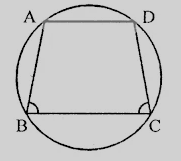"
">

# If $ABCD$ is a cyclic quadrilateral in which $AD \| BC$. Prove that $\angle B = \angle C$."

Given:

$ABCD$ is a cyclic quadrilateral in which $AD \| BC$.

To do:

We have to prove that $\angle B = \angle C$.

Solution:

$AD \| BC$

This implies,

$\angle A + \angle B = 180^o$                 (Sum of cointerior angles)

$\angle A + \angle C = 180^o$          (Opposite angles of the cyclic quadrilateral)

This implies,

$\angle A + \angle B = \angle A + \angle C$

$\Rightarrow \angle B = \angle C$

Hence proved.

Updated on: 10-Oct-2022

24 Views